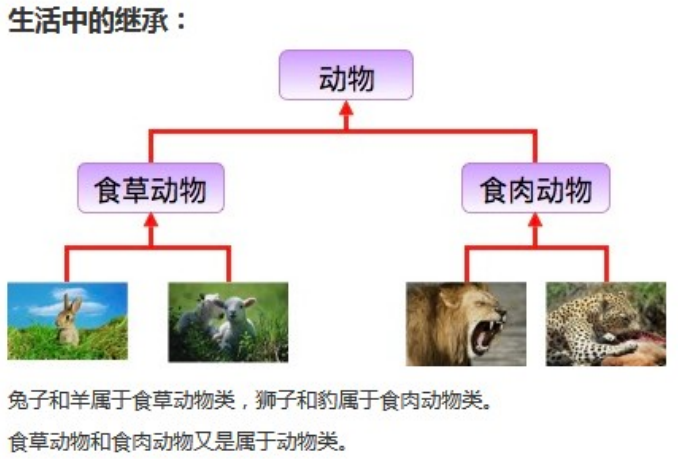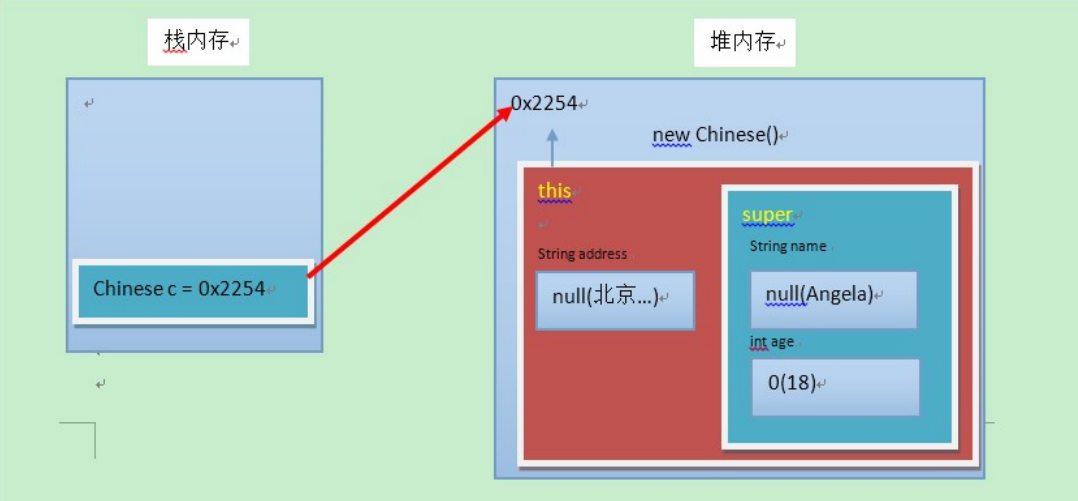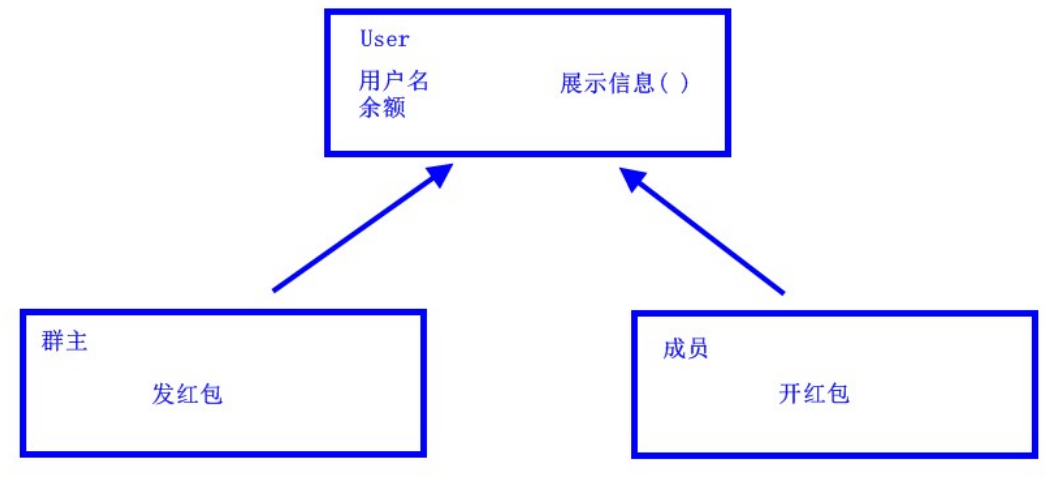#雪月书韵茶香

/

## 继承、super、this、抽象类# 第一章 继承

## 1.1 概述

### 由来### 定义

• 继承 ：就是子类继承父类的属性和行为，使得子类对象具有与父类相同的属性、相同的行为。子类可以直接访问父类中的非私有的属性和行为

### 好处

1. 提高代码的复用性。
2. 类与类之间产生了关系，是多态的前提。

## 1.2 继承的格式

``````class 父类 {
...
}
class 子类 extends 父类 {
...
}
``````

``````/*
* 定义员工类Employee，做为父类
* /

class Employee {
String name; // 定义name属性
// 定义员工的工作方法
public void work() {
System.out.println("尽心尽力地工作");
}
}
/*
* 定义讲师类Teacher 继承 员工类Employee
*/
class Teacher extends Employee {
// 定义一个打印name的方法
public void printName() {
System.out.println("name=" + name);
}
}
/*
* 定义测试类
*/
public class ExtendDemo01 {
public static void main(String[] args) {
// 创建一个讲师类对象
Teacher t = new Teacher();

// 为该员工类的name属性进行赋值
t.name = "小明";

// 调用该员工的printName()方法
t.printName(); // name = 小明

// 调用Teacher类继承来的work()方法
t.work();  // 尽心尽力地工作
}
}
``````

## 1.3 继承后的特点——成员变量

### 成员变量不重名

``````
class Fu {
// Fu中的成员变量。
int num = 5;
}
class Zi extends Fu {
// Zi中的成员变量
int num2 = 6;
// Zi中的成员方法
public void show() {
// 访问父类中的num，
System.out.println("Fu num="+num); // 继承而来，所以直接访问。
// 访问子类中的num2
System.out.println("Zi num2="+num2);
}
}
class ExtendDemo02 {
public static void main(String[] args) {
// 创建子类对象
Zi z = new Zi();
// 调用子类中的show方法
z.show();
}
}

Fu num = 5
Zi num2 = 6
``````

### 成员变量重名

``````
class Fu {
// Fu中的成员变量。
int num = 5;
}
class Zi extends Fu {
// Zi中的成员变量
int num = 6;
public void show() {
// 访问父类中的num
System.out.println("Fu num=" + num);
// 访问子类中的num
System.out.println("Zi num=" + num);
}
}
class ExtendsDemo03 {
public static void main(String[] args) {
// 创建子类对象
Zi z = new Zi();
// 调用子类中的show方法
z.show();
}
}

Fu num = 6
Zi num = 6
``````

super.父类成员变量名

``````class Zi extends Fu {
// Zi中的成员变量
int num = 6;
public void show() {
//访问父类中的num
System.out.println("Fu num=" + super.num);
//访问子类中的num
System.out.println("Zi num=" + this.num);
}
}

Fu num = 5
Zi num = 6
``````

## 1.4 继承后的特点——成员方法

### 成员方法不重名

``````class Fu{
public void show(){
System.out.println("Fu类中的show方法执行");
}
}
class Zi extends Fu{
public void show2(){
System.out.println("Zi类中的show2方法执行");
}
}
public  class ExtendsDemo04{
public static void main(String[] args) {
Zi z = new Zi();
//子类中没有show方法，但是可以找到父类方法去执行
z.show();
z.show2();
}
}
``````

## 成员方法重名 ——重写(Override)

• 方法重写 ：子类中出现与父类一模一样的方法时（返回值类型，方法名和参数列表都相同），会出现覆盖果，也称为重写或者复写。声明不变，重新实现。

``````class Fu {
public void show() {
System.out.println("Fu show");
}
}
class Zi extends Fu {
//子类重写了父类的show方法
public void show() {
System.out.println("Zi show");
}
}
public class ExtendsDemo05{
public static void main(String[] args) {
Zi z = new Zi();
// 子类中有show方法，只执行重写后的show方法
z.show();  // Zi show
}
}
``````

### 重写的应用

``````class Phone {
public void sendMessage(){
System.out.println("发短信");
}
public void call(){
System.out.println("打电话");
}
public void showNum(){
System.out.println("来电显示号码");
}
}
``````
``````//智能手机类
class NewPhone extends Phone {

//重写父类的来电显示号码功能，并增加自己的显示姓名和图片功能
public void showNum(){
//调用父类已经存在的功能使用super
super.showNum();
//增加自己特有显示姓名和图片功能
System.out.println("显示来电姓名");
System.out.println("显示头像");
}
}
public class ExtendsDemo06 {
public static void main(String[] args) {
// 创建子类对象
NewPhone np = new NewPhone()；

// 调用父类继承而来的方法
np.call();

// 调用子类重写的方法
np.showNum();
}
}
``````

## 注意事项

1. 构造方法的名字是与类名一致的。所以子类是无法继承父类构造方法的。
2. 构造方法的作用是初始化成员变量的。所以子类的初始化过程中，必须先执行父类的初始化动作。子类的构造方法中默认有一个 super() ，表示调用父类的构造方法，父类成员变量初始化后，才可以给子类使用。

``````class Fu {
private int n;
Fu(){
System.out.println("Fu()");
}
}
``````
``````class Zi extends Fu {
Zi(){
// super（），调用父类构造方法
super();
System.out.println("Zi（）");
}
}
public class ExtendsDemo07{
public static void main (String args[]){
Zi zi = new Zi();
}
}

Fu（）
Zi（）
``````

## 1.6 super 和 this

### 父类空间优先于子类对象产生### super 和 this 的含义

• super ：代表父类的存储空间标识(可以理解为父亲的引用)
• this ：代表当前对象的引用(谁调用就代表谁)

### super 和 this 的用法

this.成员变量 ‐‐ 本类的
super.成员变量 ‐‐ 父类的
this.成员方法名() ‐‐ 本类的
super.成员方法名() ‐‐ 父类的

``````class Animal {
public void eat() {
System.out.println("animal : eat");
}
}
class Cat extends Animal {
public void eat() {
System.out.println("cat : eat");
}
public void eatTest() {
this.eat();   // this  调用本类的方法
super.eat();  // super 调用父类的方法
}
}
public class ExtendsDemo08 {
public static void main(String[] args) {
Animal a = new Animal();
a.eat();
Cat c = new Cat();
c.eatTest();
}
}

animal : eat
cat : eat
animal : eat
``````
1. 访问构造方法

this(...) ‐‐ 本类的构造方法
super(...) ‐‐ 父类的构造方法

super() 和 this() 都必须是在构造方法的第一行，所以不能同时出现。

## 1.7 继承的特点

1. Java 只支持单继承，不支持多继承。
``````//一个类只能有一个父类，不可以有多个父类。
class C extends A{}  //ok
class C extends A，B... //error
``````
1. Java 支持多层继承(继承体系)。
``````class A{}
class B extends A{}
class C extends B{}
``````

1. 子类和父类是一种相对的概念。

# 第二章 抽象类

## 2.1 概述

### 定义

• 抽象方法 ： 没有方法体的方法
• 抽象类 ：包含抽象方法的类

## 2.2 abstract 使用格式

### 抽象方法

public abstract void run()；

### 抽象类

``````abstract class 类名字 {

}
``````

``````public abstract class Animal {
public abstract void run()；
}
``````

### 抽象的使用

``````public class Cat extends Animal {
public void run (){
System.out.println("小猫在墙头走~~~")；
}
}
public class CatTest {
public static void main(String[] args) {
// 创建子类对象
Cat c = new Cat();

// 调用run方法
c.run();
}
}

``````

## 2.3 注意事项

1 . 抽象类不能创建对象，如果创建，编译无法通过而报错。只能创建其非抽象子类的对象

2 . 抽象类中，可以有构造方法，是供子类创建对象时，初始化父类成员使用的。

1. 抽象类中，不一定包含抽象方法，但是有抽象方法的类必定是抽象类。

4 . 抽象类的子类，必须重写抽象父类中所有的抽象方法，否则，编译无法通过而报错。除非该子类也是抽象

# 第三章 继承的综合案例

## 3.1 综合案例：群主发普通红包

1. 群主的一笔金额，从群主余额中扣除，平均分成 n 等份，让成员领取。
2. 成员领取红包后，保存到成员余额中。

## 3.2 案例分析## 3.3 案例实现

``````  public class User {

// 成员变量
private double leftMoney; // 余额
// 构造方法
public User() { }
public User(String username, double leftMoney) {
this.leftMoney = leftMoney;
}
// get/set方法
}
}
public double getLeftMoney() {
return leftMoney;
}
public void setLeftMoney(double leftMoney) {
this.leftMoney = leftMoney;
}
// 展示信息的方法
public void show() {
System.out.println("用户名:"+ username +" , 余额为:" + leftMoney + "元");
}
}
``````

``````public class QunZhu extends User {
// 添加构造方法
public QunZhu() {
}
public QunZhu(String username, double leftMoney) {
// 通过super 调用父类构造方法
}
/*

1.获取群主余额,是否够发红包.

2.修改群主余额.
3.拆分红包.
3.1.如果能整除，那么就平均分。
3.2.如果不能整除，那么就把余数分给最后一份。
*/
public ArrayList<Double> send(int money, int count) {
// 获取群主余额
double leftMoney = getLeftMoney();
if(money > leftMoney) {
return null;
}
// 修改群主余额的
setLeftMoney(leftMoney ‐ money);

// 创建一个集合,保存等份金额
ArrayList<Double> list = new ArrayList<>();

// 扩大100倍,相当于折算成'分'为单位,避免小数运算损失精度的问题
money = money * 100;

// 每份的金额
int m = money / count;
// 不能整除的余数
int l = money % count;

// 无论是否整除,n‐1份,都是每份的等额金额
for (int i = 0; i < count ‐ 1; i++) {
// 缩小100倍,折算成 '元'
}

// 判断是否整除
if (l == 0) {
// 能整除, 最后一份金额,与之前每份金额一致
} else {
// 不能整除, 最后一份的金额,是之前每份金额+余数金额
}

// 返回集合
return list;
}
}
``````

``````public class Member extends  User {
public Member() {
}
public Member(String username, double leftMoney) {
}
// 打开红包,就是从集合中,随机取出一份,保存到自己的余额中
public void openHongbao(ArrayList<Double> list) {
// 创建Random对象
Random r = new Random();
// 随机生成一个角标
int index = r.nextInt(list.size());
// 移除一个金额
Double money = list.remove(index);
// 直接调用父类方法,设置到余额
setLeftMoney( money );
}
``````

``````public class Test {
public static void main(String[] args) {
// 创建一个群主对象
QunZhu qz = new QunZhu("群主" , 200);

// 创建一个键盘录入
Scanner sc = new Scanner();
System.out.println("请输入金额:");
int money = sc.nextInt();
System.out.println("请输入个数:");
int count = sc.nextInt();

// 发送红包
ArrayList<Double> sendList = s.send(money,count);

// 判断,如果余额不足
if(sendList == null){
System.out.println(" 余额不足...");
return;
}
// 创建三个成员
Member m = new Member();
Member m2 = new Member();
Member m3 = new Member();

// 打开红包
m.openHongbao(sendList);
m2.openHongbao(sendList);
m3.openHongbao(sendList);
// 展示信息
qz.show();
m.show();
m2.show();
m3.show();
}
}
``````